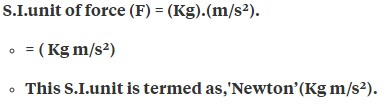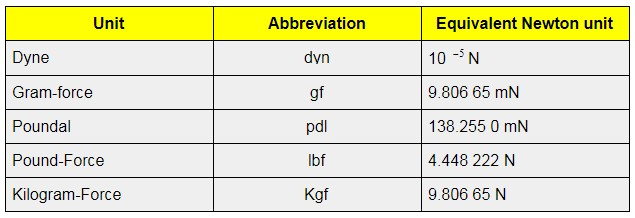Checkout JEE MAINS 2022 Question Paper Analysis : Checkout JEE MAINS 2022 Question Paper Analysis :

# Unit of Force

We all know who Isaac Newton is and it was his research that led to the establishment of the popular formula F = ma representing acceleration and mass of an object when force is applied. While students can check the article on force in detail, here we will learn about the unit of force.

Well, force is one of the quantities like pressure or energy so it has or is measured in a unit. However, force units can be different based on different systems of measurements. Let’s get into the details.

If we consider the physical dimensions, force is mostly measured by MLT-2. If we take things practically, the weight of an object is mostly taken into consideration instead of its mass. Therefore, the basic unit of force can be given as,

 F = (W/g)LT-2

where W represents the weight whereas g represents the acceleration.

The unit is also classified into two systems called gravitational system and absolute system. When force is measured in the absolute system it is independent of any gravitational force. On the other hand, when force is measured in the gravitational system, it is dependent on gravitational forces.

Apart from that, there are few other ways we can express the unit of measurement of force.

## SI Unit of Force

Internationally, many prefer to use a single system called SI unit for measuring force. The SI unit recognized for measurement of force is Newton.For your reference, in the table below we have provided the SI unit of energy and power.

 SI Unit of Energy and Power Unit of Energy Unit of Power

### Other Units of Force

When a mass is subjected to an acceleration, the force applied is called poundal and is depicted as pdl. Then we have the pound-force which is basically a gravitational unit of force. It is denoted as lbf. These are the units of measurement in the FPS system.

In the metric system, the force is measured in kilogram and is denoted by symbol kgf. However, there are also smaller measures called the cgs system. When we talk about the CGS unit of force, it is measured in dynes and is denoted as gf.

#### Converting The Units Of ForceTo know about force and types of force in detail, click on the video below.## Frequently Asked Questions – FAQs

### What is the CGS unit of force?

The CGS unit of force is dyne.

### What is the SI unit of force?

The SI unit of force is Newton.

F = (W/g)LT-2

### How is force measured

Force is measured in MLT-2.

### How is force measured in the metric system?

In the metric system, force is measured in kilograms and is denoted by the symbol kgf.

Test your Knowledge on unit of force